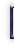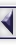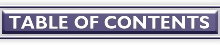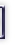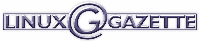...making Linux just a little more fun! Programming Bits: C# Data Types By Ariel Ortiz Ramirez

In my previous article, I introduced the C# programming language and explained how it works in the context of the Mono environment, an open-source implementation of Microsoft's .NET framework. I will now go on to some details on the data types supported by the C# programming language.

In the subsequent discussion I will use the following diagrammatic notation to represent variables and objects:The variable diagram is a cubic figure that depicts three traits (name, value and type) relevant during the compilation and execution of a program. In the von Neumann architecture tradition, we will consider a variable as a chunk of memory in which we hold a value that can be read or overwritten. The object diagram is a rounded edge rectangle that denotes an object created at runtime and allocated in a garbage collectable heap. For any object in a certain point in time, we know what type (class) it is, and the current values of its instance variables.

## Type Categories

In the C# programming language, types are divided in three categories:

• value types
• reference types
• pointer types

In a variable that holds a value type, the data itself is directly contained within the memory allotted to the variable. For example, the following code

`int x = 5;`

declares an 32-bit signed integer variable, called `x`, initialized with a value of 5. The following figure represents the corresponding variable diagram:Note how the value 5 is contained within the variable itself.

On the other hand, a variable that holds a reference type contains the address of an object stored in the heap. The following code declares a variable called `y` of type `object` which gets initialized, thanks to the `new` operator, so that it refers to a new heap allocated `object` instance (`object` is the base class of all C# types, but more of this latter).

`object y = new object();`

The corresponding variable/object diagram would be:In this case, we can observe that the "value" part of the variable diagram contains the start of an arrow that points to the referred object. This arrow represents the address of the object inside the memory heap.

Now, let us analyze what happens when we introduce two new variables and do some copying from the original variables. Assume we have the following code:

```int a = x;
object b = y; ```

The result is displayed below: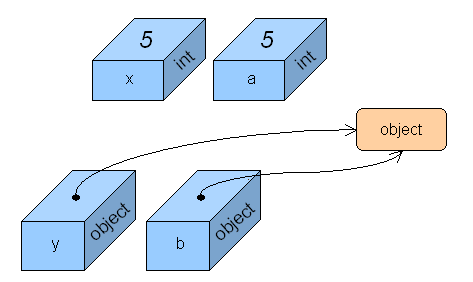As can be observed, `a` has a copy of the value of `x`. If we modify the value of one of these variables, the other variable would remain unchanged. In the case of `y` and `b`, both variables refer to the same object. If we alter the state of the object using variable `y`, then the resulting changes will be observable using variable `b`, and vice versa.

Aside from references into the heap, a reference type variable may also contain the special value `null`, which denotes a nonexistent object. Continuing with the last example, if we have the statements

```y = null;
b = null;```

then variables `y` and `b` no longer refer to any specific object, as shown below: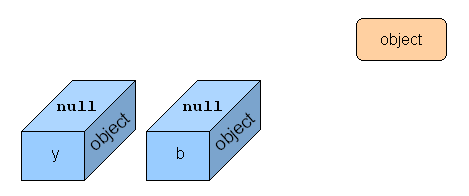As can be seen, all references to the object instance have been lost. This object has now turned into "garbage" because no other live reference to it exists. As noted before, in C# the heap is garbage collected, which means that the memory occupied by these "dead" objects is at sometime automatically disposed and recycled by the runtime system. Other languages, such as C++ and Pascal, do not have this kind of automatic memory management scheme. Programmers for these languages must explicitly free any heap allocated memory chunks that the program no longer requires. Failing to do so gives place to memory leaks, in which certain portions of memory in a program are wasted because they haven't been signaled for reuse. Experience has shown that explicit memory de-allocation is cumbersome and error prone. This is why many modern programming languages (such as Java, Python, Scheme and Smalltalk, just to name a few) also incorporate garbage collection as part of their runtime environment.

Finally, a pointer type gives you similar capabilities as those found with pointers in languages like C and C++. It is important to understand that both pointers and references actually represent memory addresses, but that's where their similarities end. References are tracked by the garbage collector, pointers are not. You can perform pointer arithmetic on pointers, but not on references. Because of the unwieldy nature associated to pointers, they can only be used in C# within code marked as `unsafe`. This is an advanced topic and I won't go deeper into this matter at this time.

## Predefined Types

C# has a rich set of predefined data types which you can use in your programs. The following figure illustrates the hierarchy of the predefined data types found in C#: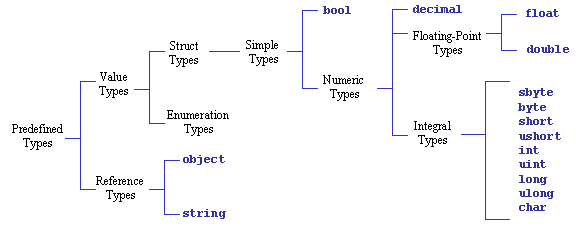Here is a brief summary of each of these types:

Type Size in
Bytes
Description
`bool` 1 Boolean value. The only valid literals are `true` and `false`.
`sbyte` 1 Signed byte integer.
`byte` 1 Unsigned byte integer.
`short` 2 Signed short integer.
`ushort` 2 Unsigned short integer.
`int` 4 Signed integer. Literals may be in decimal (default) or hexadecimal notation (with an `0x` prefix).  Examples: `26`, `0x1A`
`uint` 4 Unsigned integer. Examples: `26U`, ```0x1AU ```(mandatory `U` suffix)
`long` 8 Signed long integer. Examples: `26L`, ```0x1AL ```(mandatory `L` suffix)
`ulong` 8 Unsigned long integer. Examples: `26UL`, ```0x1AUL ```(mandatory `UL` suffix)
`char` 2 Unicode character. Example: 'A' (contained within single quotes)
`float` 4 IEEE 754 single precision floating point number. Examples: `1.2F`, `1E10F `(mandatory `F` suffix)
`double` 8 IEEE 754 double precision floating point number. Examples: `1.2`, `1E10`, `1D` (optional `D` suffix)
`decimal` 16 Numeric data type suitable for financial and monetary calculations, exact to the 28th decimal place. Example: `123.45M` (mandatory `M` suffix)
`object` 8+ Ultimate base type for both value and reference types. Has no literal representation.
`string` 20+ Immutable sequence of Unicode characters. Example: ```"hello world!\n" ```(contained within double quotes)

C#'s has a unified type system such that a value of any type can be treated as an object. Every type in C# derives, directly or indirectly, from the `object` class. Reference types are treated as objects simply by viewing them as `object` types. Value types are treated as objects by performing boxing and unboxing operations. I will go deeper into these concepts in my next article.

## Classes and Structures

C# allows you to define new reference and value types. Reference types are defined using the `class` construct, while value types are defined using `struct`. Lets see them both in action in the following program:

```struct ValType {
public int i;
public double d;
public ValType(int i, double d) {
this.i = i;
this.d = d;
}
public override string ToString() {
return "(" + i + ", " + d + ")";
}
}

class RefType {
public int i;
public double d;
public RefType(int i, double d) {
this.i = i;
this.d = d;
}
public override string ToString() {
return "(" + i + ", " + d + ")";
}
}

public class Test {
public static void Main (string[] args) {

// PART 1
ValType v1;
RefType r1;
v1 = new ValType(3, 4.2);
r1 = new RefType(4, 5.1);
System.Console.WriteLine("PART 1");
System.Console.WriteLine("v1 = " + v1);
System.Console.WriteLine("r1 = " + r1);

// PART 2
ValType v2;
RefType r2;
v2 = v1;
r2 = r1;
v2.i++; v2.d++;
r2.i++; r2.d++;
System.Console.WriteLine("PART 2");
System.Console.WriteLine("v1 = " + v1);
System.Console.WriteLine("r1 = " + r1);
}
}```

First we have the structure `ValType`. It defines two instance variables, `i` and `d` of type `int` and `double`, respectively. They are declared as `public`, which means they can be accessed from any part of the program where this structure is visible. The structure defines a constructor, which has the same name as the structure itself and, contrary to method definitions, has no return type. Our constructor is in charge of the initialization of the two instance variables. The keyword `this` is used here to obtain a reference to the instance being created and has to be used explicitly in order to avoid the ambiguity generated when a parameter name clashes with the an instance variable name. The structure also defines a method called `ToString`, that returns the external representation of a structure instance as a string of characters. This method overrides the `ToString` method (thus the use of the `override` modifier) defined in this structure's base type (the `object` class). The body of this method uses the string concatenation operator (+) to generate a string of the form "(i, d)", where i and d represent the current value of those instance variables, and finally returns the expected result.

As can be observed, the `RefType` class has basically the same code as `ValType`. Let us examine the runtime behavior of variables declared using both types so we can further understand their differences. The `Test` class has a `Main` method that establishes the program entry point. In the first part of the program (marked with the "PART 1" comment) we have one value type variable and one reference type variable. This is how they look after the assignments: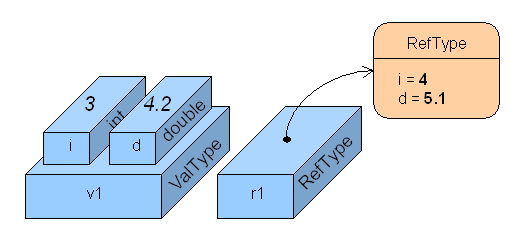The value type variable, `v1`, has its instance variables contained within the variable itself. The `new` operator used in the assignment

`v1 = new ValType(3, 4.2);`

does not allocate any memory in the heap as we've learned from other languages. Because `ValType` is a value type, the new operator is only used in this context to call its constructor and this way initialize the instance variables. Because `v1` is a local variable, it's actually stored as part of the method's activation record (stack frame), and it exists just because it's declared.

Objects referred by reference type variables have to be created explicitly at some point in the program. In the assignment

`    r1 = new RefType(4, 5.1);`

the `new` operator does the expected dynamic memory allocation because in this case `RefType` is a reference type. The corresponding constructor gets called immediately afterwards. Variable `v2` is also stored in the method's activation record (because it's also a local variable) but it's just big enough to hold the reference (address) of the newly created instance. All the instance's data is in fact stored in the heap.

Now lets check what happens when the second part of the program (marked after the "PART 2" comment) is executed. Two new variable are introduced and they are assigned the values of the two original ones. Then, each of the instance variables of the new variables are incremented by one (using the `++` operator).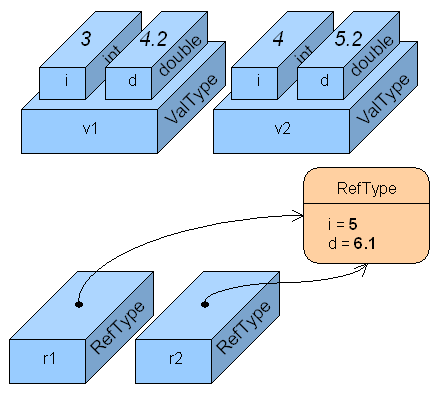When `v1` is copied into `v2`, each individual instance variable of the source is copied individually into the destination, thus producing totally independent values. So any modification done over `v2` doesn't affect `v1` at all. This is not so with `r1` and `r2` in which only the reference (address) is copied. Any change to the object referred by `r2` is immediately seen by `r1`, because they both refer in fact to the same object.

If you check the type hierarchy diagram above, you will notice that simple data types such as `int`, `bool` and `char` are actually `struct` value types, while `object` and `string` are `class` reference types.

If you want to compile and run the source code of the above example, type at the Linux shell prompt:

`mcs varsexample.cs`
`mono varsexample.exe`

The output should be:

```PART 1
v1 = (3, 4.2)
r1 = (4, 5.1)
PART 2
v1 = (3, 4.2)
r1 = (5, 6.1)```

## Resources

http://www.go-mono.com/
The official Mono home page. You can find here the download and install instructions for the Mono platform. It includes a C# compiler, a runtime environment, and a class library.
http://msdn.microsoft.com/library/default.asp?url=/library/en-us/cscon/html/vcoriCStartPage.asp
General information on the C# programming language.